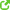# Solving Einstein's Equation with Cosmological Constant: De Sitter Space

• binbagsss
No, they correspond to different homeomorphisms from the same open subset of the manifold (in this case, all of de Sitter spacetime, which is an open manifold) to open subsets of 4-dimensional Euclidean space. The open subset of Euclidean space that is used can be the same for different charts, or different. Even if the two open subsets (of the manifold and of Euclidean space) are the same, that does not prevent there from being multiple possible homeomorphisms between them; the different charts in this case would map the same point in de Sitter spacetime to different 4-tuples of real numbers, but the range of 4-tuples covered would still be the same forf

#### binbagsss

I'm looking at solutions to Einsten's equation with the cosmological constant included ,##G_{uv}=- 8 \pi GT_{uv} - \lambda g_{uv} ##, with the FRW metric at hand - i.e, ## R_{uv} ## has been computedfrom this metric.

I have a question from these notes...http://arxiv.org/abs/gr-qc/9712019
they give the solution for ##\lambda <0 ##(and the only case of ##k=1##) as ## a= \sqrt{\frac{-3}{\lambda}}sin(\sqrt\frac{-\lambda}{3}t) ##

##\lambda >0 ##, for ##k=-1,0,1## are respectively:

## a= \sqrt{\frac{3}{\lambda}}sinh(\sqrt/frac{\lambda}{3}t) ##
## a \propto exp (\pm \sqrt{/frac{\lambda}{3}}t) ##
## a= \sqrt{\frac{3}{\lambda}}cosh(\sqrt\frac{\lambda}{3}t) ##

It then says that the ##k=-1,0,1 ##and ##\lambda >0 ## are all the same solutions, just in different coordinates.

Question:

The FRW metric is given in spherical polar coordinates. So when we solve for the solutions, how is it that they are in different coordinates? Would it be the use of various integration substitutions to solve, and what's given here, is the most easiest substitution that solves for each k, and so this easiest substituion has differed for each case?

Last edited:
The FRW metric is given in spherical polar coordinates. So when we solve for the solutions, how is it that they are in different coordinates?

Because "spherical polar coordinates" is not a single coordinate chart. It's a whole family of charts, which can differ by how the remaining two coordinates, usually called ##t## and ##r##, are determined. This amounts to different ways in which spacetime is split up into space and time. In the case of de Sitter spacetime, there are different ways of doing this that correspond to all three values of k, i.e., -1, 0, and 1. All of these coordinate charts describe the same spacetime geometry (i.e., the same solution). The different charts give rise to different expressions for the scale factor as a function of the time coordinate because of the different space-time splitting.

Because "spherical polar coordinates" is not a single coordinate chart. It's a whole family of charts, which can differ by how the remaining two coordinates, usually called ##t## and ##r##, are determined. This amounts to different ways in which spacetime is split up into space and time. In the case of de Sitter spacetime, there are different ways of doing this that correspond to all three values of k, i.e., -1, 0, and 1. All of these coordinate charts describe the same spacetime geometry (i.e., the same solution). The different charts give rise to different expressions for the scale factor as a function of the time coordinate because of the different space-time splitting.

Thanks for your reply, I'm being introduced to GR with as little geometry as possible, and so have not covered coordinate charts. I've had a quick google around and see it is defined as a homeomorphism from a open subset on the manifold to an open subset in Euclidean space,

so when we say the different de-sitter spaces correspond to different coordinate charts, in effect then, have they been comptuted on different subsets of the manifold?

I'm also having struggling how to picture this explicitly, i.e- spherical coordinates etc, is a 'usual' coordinate transformation, so what I know before coordinate charts, then defined as a global chart?

Thanks

when we say the different de-sitter spaces correspond to different coordinate charts, in effect then, have they been computed on different subsets of the manifold?

No, they correspond to different homeomorphisms from the same open subset of the manifold (in this case, all of de Sitter spacetime, which is an open manifold) to open subsets of 4-dimensional Euclidean space. The open subset of Euclidean space that is used can be the same for different charts, or different. Even if the two open subsets (of the manifold and of Euclidean space) are the same, that does not prevent there from being multiple possible homeomorphisms between them; the different charts in this case would map the same point in de Sitter spacetime to different 4-tuples of real numbers, but the range of 4-tuples covered would still be the same for both charts.